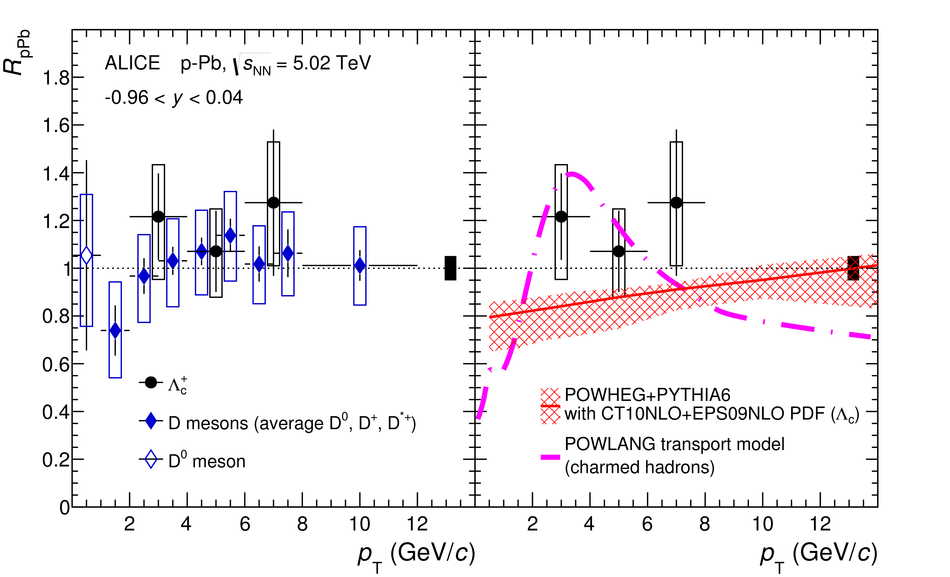# Figure 12

 The nuclear modification factor \RpPb of prompt \Lcplus~baryons in \pPb collisions at \sqrtsNN = 5.02 TeV as a function of \pT compared to that of D mesons (average of \Dzero, \Dplus and \Dstar in the range $1 < \pt < 12$~\gevc, and \Dzero in the range $0 < \pt < 1$~\gevc)~ (left panel) and to model calculations (right panel). The predictions for the comparison are the \Lcplus~\RpPb~from the {\sc powheg} event generator~ with EPS09NLO parameterisation of the nuclear modification of the PDFs~ and the charm-hadron \RpPb from the POWLANG transport model~ assuming a QGP is formed in \pPb collisions.